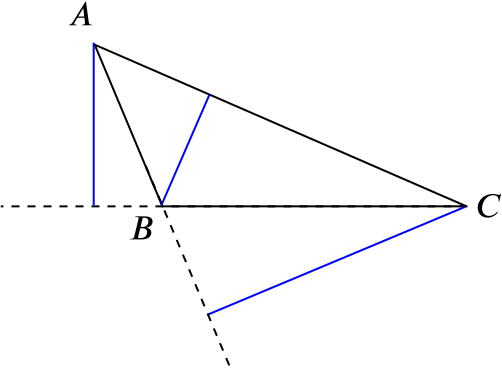# Altitude of triangleA triangle $ABC$ has 3 altitudes. These are the perpendicular line segments from $A$ to the side $BC$ (possibly extended), and similarly from $B$ to $CA$, and from $C$ to $AB$.

The altitudes are shown in blue in this diagram.